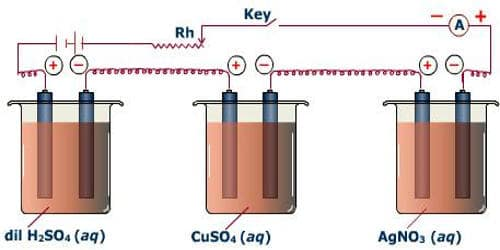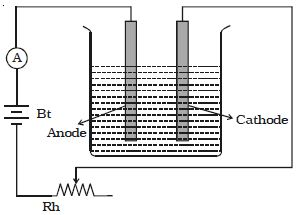# Verification of Faraday’s First Law of Electrolysis

Verification of Faraday’s first law of electrolysis

First Law: The mass of a substance liberated at an electrode is directly proportional to the charge passing through the electrolyte. The mass of a substance altered at an electrode during electrolysis is directly proportional to the quantity of electricity transferred at that electrode. Quantity of electricity refers to the quantity of electrical charge, typically measured in coulomb.

A battery, a rheostat, a key, and an ammeter are connected in series to an electrolytic cell (Figure). The cathode is cleaned, dried, weighed, and then inserted in the cell. A current I1 is passed for a time t. The current is measured by the ammeter. The cathode is taken out, washed, dried, and weighed again. Hence the mass m1 of the substance deposited is obtained.The cathode is reinserted in the cell and a different current I2 is passed for the same time t. The mass m2 of the deposit is obtained. It is found that –

m1/m2 = I1/I2

so, m α I … … … (1)

The electrochemical equivalent of a substance is defined as the mass of substance liberated in electrolysis when the one-coulomb charge is passed through the electrolyte. The experiment is repeated for the same current I but for different times t1 and t2. If the masses of the deposits are m3 and m4 respectively, it is found that

m3/ m4 = t1/ t2

so, m α t … … … (2)

From relations (1) and (2)

m α It or m α q Thus, the first law is verified.

Verify Faraday’s First law by passing different quantities of electricity in the same solution and calculating the ratio of masses of the formed materials at the cathode or dissolved from the anode and comparing these ratios of the passed quantities of electricity.

Observation: Increasing the mass of substance at the cathode by increasing of quantity of electricity passed in solution, we find that m ∝ Q.

Conclusion: the quantity of material formed or consumed at any electrode is directly proportional to the quantity of electricity that passes in the electrolytic (solution or molten) that is called Faraday’s First law.